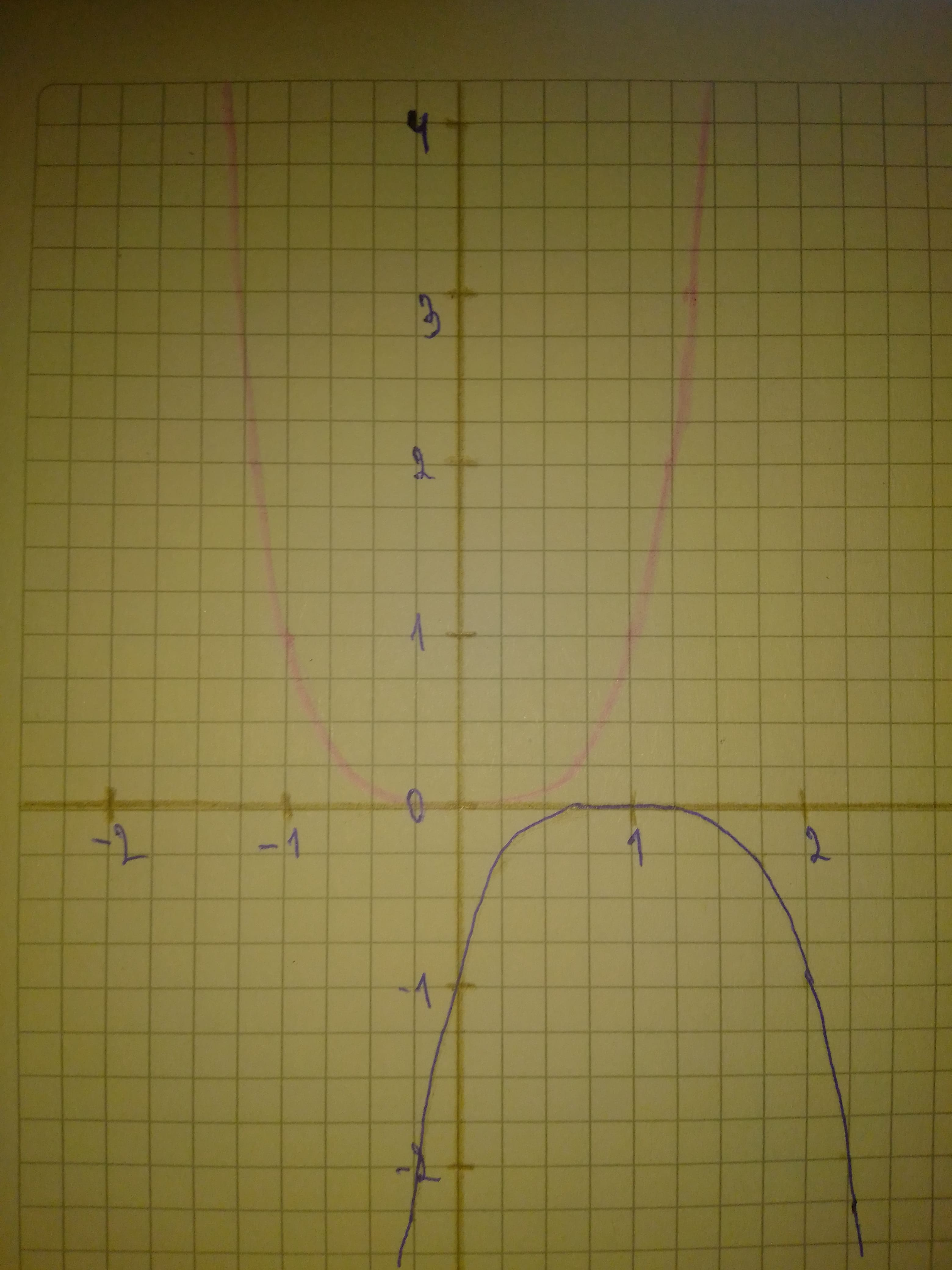# Sketch a graph of the function. Use transformations of functions whenever possible. f(x)=-(x-1)^{4}pancha3 2021-03-18 Answered
Sketch a graph of the function. Use transformations of functions whenever possible. $f\left(x\right)=-{\left(x-1\right)}^{4}$
You can still ask an expert for help

• Questions are typically answered in as fast as 30 minutes

Solve your problem for the price of one coffee

• Math expert for every subject
• Pay only if we can solve itwheezym

Step 1
First graph function $y={x}^{4}$
Then, to obtain graph of function $f\left(x\right)=-{\left(x-1\right)}^{4}$, do following transformations:
-shift 1 unit to the right
-reflect over x-axis
On graph:
Red - $y={x}^{4}$
Blue - $f\left(x\right)=-{\left(x-1\right)}^{4}$
Step 2